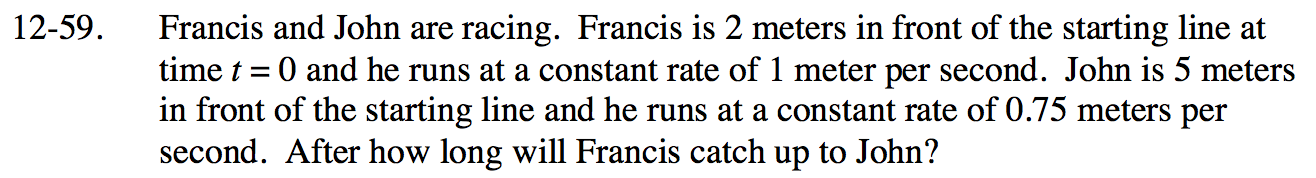### Home > INT2 > Chapter 12 > Lesson 12.2.1 > Problem12-59

12-59.

Francis and John are racing. Francis is 2 meters in front of the starting line at time t = 0 and he runs at a constant rate of 1 meter per second. John is 5 meters in front of the starting line and he runs at a constant rate of 0.75 meters per second. After how long will Francis catch up to John? Homework Help ✎Francis: d = 1t + 2

where d = distance from starting line (m) t = time (seconds)

Write the same type of equation for John.

What must be true about the two equations if they are at the same distance?

Use the Equal Values method to solve for t.

12 seconds